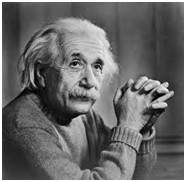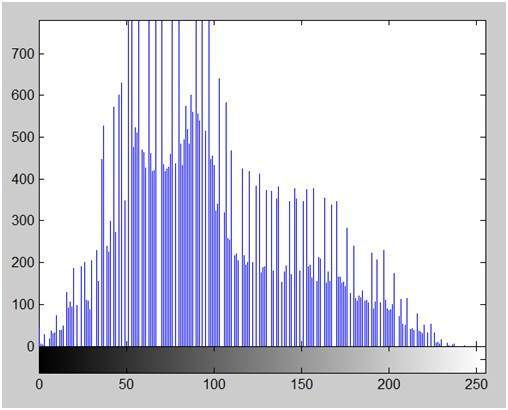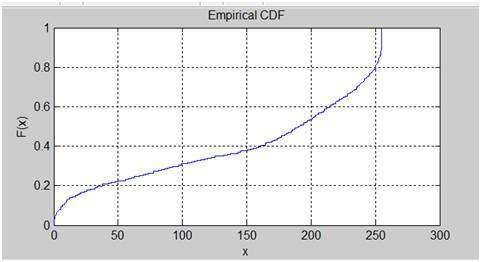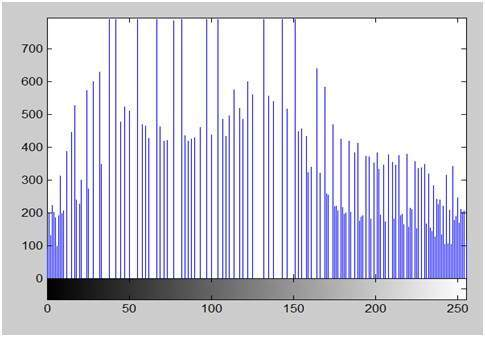# Histogram Equalization

We have already seen that contrast can be increased using histogram stretching. In this tutorial we will see that how histogram equalization can be used to enhance contrast.

Before performing histogram equalization, you must know two important concepts used in equalizing histograms. These two concepts are known as PMF and CDF.

They are discussed in our tutorial of PMF and CDF. Please visit them in order to successfully grasp the concept of histogram equalization.

## Histogram Equalization:

Histogram equalization is used to enhance contrast. It is not necessary that contrast will always be increase in this. There may be some cases were histogram equalization can be worse. In that cases the contrast is decreased.

Lets start histogram equalization by taking this image below as a simple image.

Image### Histogram of this image:

The histogram of this image has been shown below.Now we will perform histogram equalization to it.

### PMF:

First we have to calculate the PMF (probability mass function) of all the pixels in this image. If you donot know how to calculate PMF, please visit our tutorial of PMF calculation.

### CDF:

Our next step involves calculation of CDF (cumulative distributive function). Again if you donot know how to calculate CDF , please visit our tutorial of CDF calculation.

### Calculate CDF according to gray levels

Lets for instance consider this , that the CDF calculated in the second step looks like this.

Gray Level ValueCDF
00.11
10.22
20.55
30.66
40.77
50.88
60.99
71

Then in this step you will multiply the CDF value with (Gray levels (minus) 1) .

Considering we have an 3 bpp image. Then number of levels we have are 8. And 1 subtracts 8 is 7. So we multiply CDF by 7. Here what we got after multiplying.

Gray Level ValueCDFCDF * (Levels-1)
00.110
10.221
20.553
30.664
40.775
50.886
60.996
717

Now we have is the last step , in which we have to map the new gray level values into number of pixels.

Lets assume our old gray levels values has these number of pixels.

Gray Level ValueFrequency
02
14
26
38
410
512
614
716

Now if we map our new values to , then this is what we got.

Gray Level ValueNew Gray Level ValueFrequency
002
114
236
348
4510
5612
6614
7716

Now map these new values you are onto histogram , and you are done.

Lets apply this technique to our original image. After applying we got the following image and its following histogram.

### Histogram Equalization Image### Cumulative Distributive function of this image### Histogram Equalization histogram### Comparing both the histograms and images### Conclusion

As you can clearly see from the images that the new image contrast has been enhanced and its histogram has also been equalized. There is also one important thing to be note here that during histogram equalization the overall shape of the histogram changes, where as in histogram stretching the overall shape of histogram remains same.